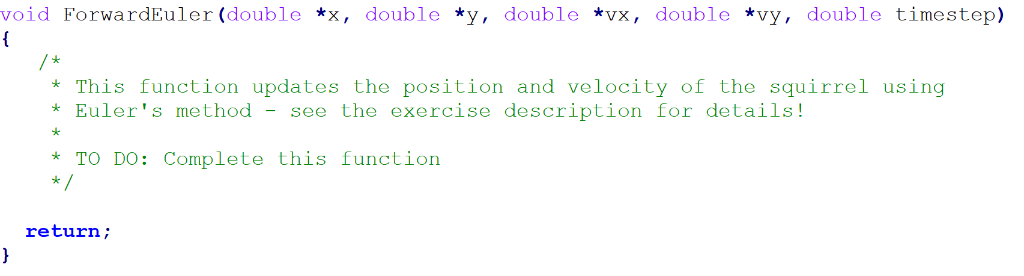# how to use c to wirte a program about euler 39 s method for a parabolic trajectory h 5154994

How to use C to wirte a program about Euler&#39;s method for a parabolic trajectory?

We update the position based on the velocity and how much time has passed:

X_t+1 = X_t + (Vx_t * time_step)

Y_t+1 = Y_t + ( Vy_t * time_step)

That is, the position at the next time instant (t+1) is just the current position (at time t) plus the velocity multiplied by the amount of time that has gone by – both for the X and Y components of position.

We also need to update the velocity

– The horizontal velocity remains constant, so

Vx_t+1 = Vx_t

(The horizontal velocity at the next time instant is just the same as the current horizontal velocity)

Vy_t+1 = Vy_t – (g*time_step)

(The vertical velocity decreases over time by an amount that is given by the acceleration of gravity &#39;g&#39; multiplied by the time step).void ForwardEuler (double *x, double *y, double *vx, double *vy, double timestep) This function updates the position and velocity of the squirrel using Euler&#39;s method – see the exercise description for details! TO DO: Complete this function return;